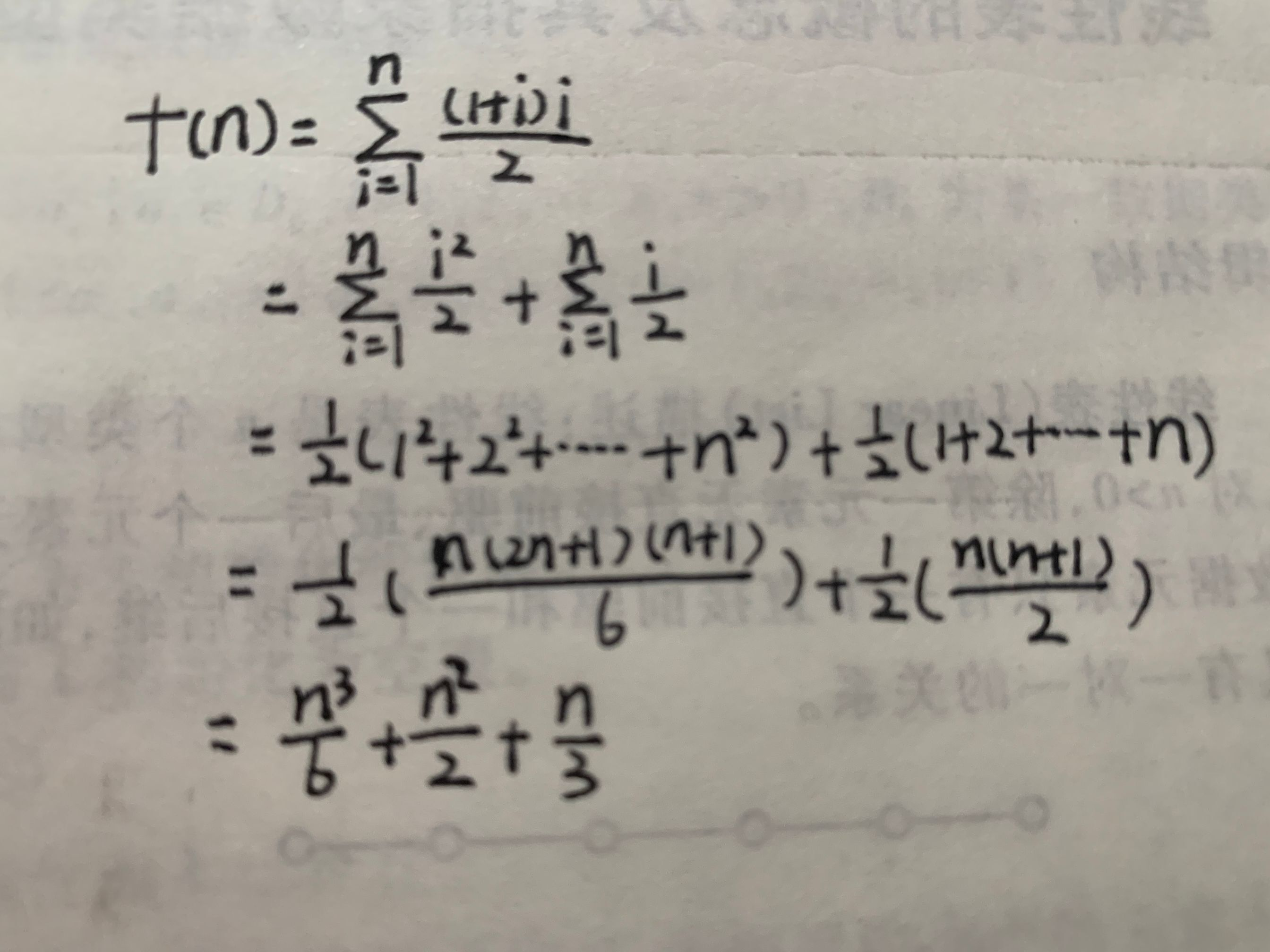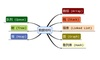• 小白学习数据结构 对于频度计算十分百思不得其解 查了很多资料感觉自己也还是不能理解，求大佬帮忙解释一下： 1. for (i=0;i;i++) 1. for (j=0;j;j++) { 1. C[i][j]=0; 1. for (k=0;k;k++) 1. C[i][j...c语言
• ## 计算语句频度

千次阅读 多人点赞 2020-08-06 23:16:01
时间频度 一个算法执行所耗费的时间，从理论上是不能算出来的，必须上机运行测试才能知道。但我们不可能也没有必要对每个算法都上机测试，只需知道哪个...一个算法中的语句执行次数称为语句频度或时间频度。记为T(n)。
这些数据结构题集（严蔚敏）书上的题，这些是我做题的笔记

语句频度 T(n)，又被称为时间频度，指的是该语句重复执行的次数
第一题
int i = 1;
int k = 0;
int n = 10;
while(i <= n-1){
k += 10 * i;  /*计算该语句频度*/
i++;
}


while 循环了多少次，就是该语句的频度 while 执行一次 i 自增 1 ，当 i>n-1 时退出，就是当 i=n 时退出 while ，i 一开始为 1，所以 while 总共循环了 n-1 次; 频度 ：n-1

第二题
int i = 1;
int k = 0;
int n = 10;
do{
k += 10 * i;  /*计算该语句频度*/
i++;
}while(i <= n-1);


循环了多少次，就是该语句的频度 循环体执行一次 i 自增 1 ，当 i>n-1 时退出，就是当 i=n 时退出循环体，i 一开始为 1 ，所以循环体总共循环了 n-1 次; 频度 ：n-1

第三题
int i = 1;
int k = 0;
int n = 10;
while(i <= n-1){
i++;
k += 10 * i;  /*计算该语句频度*/
}


循环了多少次，就是该语句的频度 循环体执行一次 i 自增 1 ，当 i>n-1 时退出，就是当 i=n 时退出循环体，i 一开始为 1 ，所以循环体总共循环了 n-1 次; 频度 ：n-1

第四题
int k = 0;
int n = 10;
for(int i = 1; i <= n; i++){
for(int j = i; j<=n; j++){
k++;    /*计算该语句频度*/
}
}


第一层循环 n 次 第二层循环 n+(n-1)+(n-2)+…+2+1 = n(n+1)/2 ,所以 k++ 执行了 n(n+1)/2 次 频度 ：n(n+1)/2

第五题
int n = 100;
int i = 0;
while(n >= (i+1)*(i+1)){
i++;    	/*计算该语句频度*/
}


循环体执行 floor(sqrt(n)) 次 频度:

⌊

n

⌋

\lfloor \sqrt{n}\rfloor

第六题
int x = 0;
int i,j,k;
int n = 10;
for(i = 1; i <= n; i++) {
for(j = 1; j <= i ; j++) {
for(k = 1; k <= j; k++) {
x += 1;   /*计算该语句频度*/
}
}
}


第一层 n 第二层 1+2+3+4+…+n 第三层 1+(1+2)+(1+2+3)+(1+2+3+4)+…+(1+2+3+4+…+n) = n(n+1)(2n+3)/12 频度：n(n+1)(2n+3)/12

第七题
int i = 1;
int j = 0;
int n = 10;
while(i+j <= n){
if(i > j)/*计算该语句频度*/ j++;
else i++;
}


注意：if 语句不管真假都会判断一次，所以循环了多少次就判断了多少次 if 语句 当 i+j=n+1 时退出循环 所以循环次数为 (n+1)-1 = n 所以频度为 n

第八题
int x = 91;
int y = 100;
while(y > 0){
if(x > 100){  /*计算该判断语句频度*/
x -= 10;
y--;
}else{
x++;
}
}


看 while 循环体中的 if 语句频度就看 while 循环次数 开始 x=91 ,循环了 10 次，每次都执行 else ，直到 x=101 当 x=101，循环了 1 次，if 条件成立，x 又变成了 91 ，而 y=99; while 循环还没退出之前都是按照这规律循环，直到 y=0 退出 while ，一共重复了 100 遍上面的规律，每次 11 次循环， 所以该语句频度为 100*11 = 1100

注意
int k = 1;
for(int i = 1; i <= n ;i++){  //(1)
k++;     //(2)
}

(1)语句频度是n+1
i 变量在第一个 for 循环中，从取 i = 0 开始执行，直到i=n时为止，至此，i 执行了n次。加上最后i=n+1跳出循环的判断，故，频度共n+1 次；
(2)语句频度是n
当 i = n+1时跳出循环，所以里面的循环体一共执行了 n 次0

时间复杂度
简单的说，就是保留语句频度的最高次幂，并且把系数去掉。 如

T

(

n

)

=

2

n

2

+

n

+

1

=

O

(

n

2

)

T(n)=2n^2+n+1=O(n^2)


展开全文c语言
• 语句频度。 for( i = 1 ; i <= n ; i++ ) for( j = 1 ; j <= i ; j++ ) for( k = 1 ; k <= j ; k++) x = x + 1; (出自：《数据结构——用C语言描述》(第二版) 耿国华著） 首先我们看另一个题目...
题目
计算下列程序段种x = x + 1;的语句频度。
for( i = 1 ; i <= n ; i++ )
for( j = 1 ; j <= i ; j++ )
for( k = 1 ; k <= j ; k++)
x = x + 1;

(出自：《数据结构——用C语言描述》(第二版) 耿国华著）

首先我们看另一个题目：执行下面程序，求语句S的执行次数。
for( i = 1 ; i <= n ; i++ )				//设为语句1
for( j = 1 ; j <= i ; j++ )			//设为语句2
S;

分析：我们设S执行的次数为f(n). 当n = 1时，语句1执行一次，语句二也执行一次，所以f(n) = 1; 当n = 2时，语句1执行两次，当n为1时语句2执行一次，n为2时语句2执行两次，所以f(n) = 1 + 2 = 3; 当n = 3时，语句1执行三次，当语句1执行第一次时语句2执行一次，当语句1执行第二次时语句2执行两次，当语句1执行第三次时语句2执行三次，所以f(n) = 1 + 2 + 3 = 6; … 当n = n时，语句1执行n次，当语句1执行第一次时语句2执行一次，当语句1执行第二次时语句2执行两次，当语句1执行第三次时语句2执行三次，…，当语句1执行第n次时语句2执行n次，所以f(n) = 1 + 2 + 3 +…+ n = n*(n+1)/2;

现在我们再来看最开始的题目： 因为这是一个三重循环，且每一层次的循环次数都在发生改变，所以一个循环一个循环的数是不太现实的。所以我们可以采用整体法，我们可以先将
for( j = 1;j <= i; j++)
for( k =1;k <=j;k++)
x = x + 1;

看作一个整体，由第二题我们可知 x = x + 1;在这个整体中执行了i * ( i + 1)/2次，这是我们就可以将这个整体看作是上一题中的语句2，结果发现最后的结果还是需要累加来求出，于是我们得出如下公式：所以最终得出的结果就是 f(n) = (n+2)*(n+1)*n/6
展开全文• ## 语句频度

千次阅读 多人点赞 2020-04-28 10:25:19
数据结构与算法的第一章课程介绍我们的王炸—“算法”时，讲到语句频度计算，刚开始完全摸不着头脑，短短几分钟的讲解，我“李姐”了好几个小时，最终弄明白后，觉得不是它太难了，而是自己ben了。 请看题： ...
在数据结构与算法的第一章课程介绍我们的王炸—“算法”时，讲到语句频度的计算，刚开始完全摸不着头脑，短短几分钟的讲解，我“李姐”了好几个小时，最终弄明白后，觉得不是它太难了，而是自己ben了。 请看题：
  语句                                                  语句频度
for(i=0;i<n;i++){(1)                                    n＋1
for (j=0;j<n;j++){(2)                               n(n+1)/n²+n
c[i][j]=0;(3)                                   n2
for(k=0;k<n;k++){(4)                            n3＋n2/n*n*(n+1)
c[i][j]=c[i][j]+a[i][k]*b[k][j];(5)         n3
}
}
}

我给每层都编了号，（1）（2）…，方便解说 (1) 不看里面的循环，单独看（1），当i范围在0~[n-1]时，（1）执行了n次，但i=n时还执行了一次（判断后跳出循环），所以是n+1次。
(2) 在（1）的基础上，（1）i的范围在0~[n-1]时，（1）执行了n次；但在（1）执行一次时，（2）每一次都循环了n+1次；当的i=n时，跳出循环，这时（2）不执行；所以就是n(n+1)次
(3) 在（1）（2）的基础上，当i，j范围都在0 ~ [n-1]时，（3）才执行；当j=0 ~ [n-1]时，（2）循环了n²次，所以（3）的频度为n²次
(4) 跟（1）（2）结合，单独的（4）循环了（n+1次），所以（4）的频率为nn(n+1)次
(5) 同（3），i，j，k的范围都在0~[n-1]时，（5）才执行，所以（5）的频度是n³次
如果还是有点懵，到浏览器控制台输出一下会更好理解一些
展开全文• 以下程序段中语句“x++”的语句频度为： 一、解题思路 1、首先，这道题目是三层for循环嵌套，一般我们的思路是从里向外往出推结果； 2、其次，我们先观察最里边的一层循环，其变量为k，循环次数是由变量j决定的。...
以下程序段中语句“x++”的语句频度为：一、解题思路
1、首先，这道题目是三层for循环嵌套，一般我们的思路是从里向外往出推结果；
2、其次，我们先观察最里边的一层循环，其变量为k，循环次数是由变量j决定的。并且由于k的初始值是1，结束条件为j，所以第三层循环语句的执行次数便为：j次；
3、然后，我们再观察中间层循环，其变量为j，同样其循环次数也不是一个固定的值，而是由变量i决定的。因为j的初始条件为1，结束条件为i，所以里边两层的循环从次数为从1到i的求和，通过求和公式得到结果为：i*(i+1)/2次；
4、最后，我们观察最外层循环，其变量为i，循环次数是由变量n决定的。由于i的初始值为1，结束条件为n，所以从最外层到最里层的总的循环次数为对i*(i+1)/2求n项和，更具求和公式得计算得出总的语句执行次数为：n(n+1)(n+2)/6次。
二、解题步骤
如下图所示：综上所述，答案为：n(n+1)(n+2)/6次。

总结
1、当多重循环嵌套时，一定要考虑每一层之间的逻辑关系，要看清楚变量所对应的初始条件和结束条件；当初始条件和结束条件发生改变时，计算结果也会大不一样； 2、i^2的求和公式的结果为：n(n+1)(2n+1)/6次。
展开全文• 计算 算法的时间复杂度 ，先要掌握什么是算法的频度 算法频度就是基本操作执行次数的总和 （f(n) n是执行问题的规模 ） 再对整个算法的频次进行以下操作： 去掉常数项 只保留最高阶项 这就是时间复杂度 T(n) ，...
• 数据结构初学 语句频度 需要用到平方和公式来求解的语句频度计算
• s=0; for(i=1;i;i++) for(j=0;j;j++) s+=j; 求出语句执行频度c语言
• 数据结构中，频度是指一个定义变量在它的函数中，并且是它在执行到该段语句为止时，这个定义变量在函数总共执行基本操作的次数。 含义：在函数总共执行基本操作的次数 下函数中各行频度n的计算： for(i=0;i<n;i...c语言
• 学习算法的时间复杂度的渐进表示时所遇到的一个例子：计算多层for循环嵌套时的语句频度语句频度即执行最多的语句（基本语句）重复执行的次数。 for(int i=1;i<=n;i++) for(int j=1;j<=i;j++) for(int k...算法
• 数据结构理论知识：第一章 绪论1.数据：所有能被计算机识别，储存和处理的符号的集合(包括数字，字符，声音，图像等信息)2.数据结构：数据中的一个“个体”，具体完整的实际意义(又称元素，顶点，记录等)，是数据的...
• 数据结构知识点总结,个人整理版,期末宝典系列第1章绪论内容提要：◆数据结构研究的内容。针对非数值计算的程序设计问题，研究计算机的操作对象以及它们之间的关系和操作。数据结构涵盖的内容：◆基本概念：数据、...
• 在学习具体的数据结构和算法之前，每一位初学者都要掌握一个技能，即善于运用时间复杂度和空间复杂度来衡量一个算法的运行效率。 通过算法所编写出的程序的运行效率。程序的运行效率具体可以从 2 个方面衡量，分别...算法 c++
• 一个算法中的语句执行次数称为语句频度或时间频度。记为T(n)。 二、时间频度特点 1、举例说明-忽略常数项 示例如下图 结论 1）、2n+20 和 2n 随着n 变大，执行曲线无限接近, 20可以忽略 2）、3n+10 和 3n 随着n ...
• 数据结构(C语言版)》复习重点在二、三、六、七、九、十章，考试内容两大类：概念，算法，自从计算机专业课统考以后，专业课考试...试确定下列各程序段中前置以记号@的语句频度：(1) i=1 k=0while(i<=n-1)@ ...
• 2. 时间复杂度和空间复杂度的定义，常用计算语句频度来估算算法的时间复杂度。3.线性表的逻辑结构，是指线性表的数据元素间存在着线性关系。主要是指：除第一及最后一个元素外，每个结点都只有一个前趋和只有一个...
• 2：计算方法：时间复杂度就是一个算法中的语句执行次数最多的一个。 1.一个算法执行所耗费的时间，从理论上是不能算出来的，必须上机运行测试才能知道。但我们不可能也没有必要对每个算法都上机测试，只需知道哪个...
• 一个算法中语句的执行次数称为语句频度或时间频度，记为T(n). 实例：计算1~100的和。 注：第一种方式，T(n)=n+1，其中+1，是最后一次对条件判断，不成立然后退出循环。 特点： 1、忽略常数项 结论： 1）2n + 20 和 ...
• 上次发操作系统笔记，很快浏览上万，这次数据结构比上次硬核的多哦，同样的会发超硬核代码，关注吧。
• 则其执行频度为 O（n^2) 例2、 解析： 注释解释： i值取1，j值取1，k值取1，执行次数1. i值取2，j值取1，2，k值分别取1、1，2，执行次数为1+2=3. i值取3，j值取1，2，3.k值取分别为1、1，2、1，2，3.执行次数为1+2...
• 其中的f(n)一般是算法中频度最大的语句频度。 主要用算法时间复杂度的数量级(即算法的渐近时间复杂度)评价一个算法的时间性能。 常见的时间复杂度按数量级递增排列依次为：常数0(1)、对数阶0(log 2 n)、线形阶0(n)、...算法
• ## 数据结构中的时间复杂度的计算

万次阅读 多人点赞 2017-09-07 18:51:32
时间复杂度或称时间复杂性，又称计算复杂度，她说是算法有效的度量之一，时间复杂度是一个算法运行时间的相对度量，一个算法的运行时间长短，它大致等于执行一种简单操作所（赋值、比较、计算、转向、返回、输入和...算法
• 1、数据结构是带有结构的数据元的集合，一般包括三个方面的内容：数据的存储结构，数据的运算，数据的逻辑结构 2、算法一定要有输出，算法不一定要有输入，算法中的每条指定含义必须要明确，算法中的每一条指定的...
• 今天早上突然想总结一下数据结构的时间复杂度的知识。 之前学了很多遍，但是一直没有总结，所以之前参考了Algorithm还有清华大学出版的那个数据结构书，今天早上花了几个小时好好的总结一下，也送给三班的同学们。 ...
• 数据、数据元素、数据结构、数据类型、抽象数据类型、逻辑结构和存储结构、算法及其设计原则、算法五个要素、 问题的规模、语句频度、时间复杂度、空间复杂度。 2.理解算法五个要素的确切含义 3.掌握计算语句频度和...
• 第一章 ...熟悉C语言的书写规范，理解算法的5个要素的确切含义，即有穷性、确定性、可行性及有输入、有输出，从而掌握计算语句频度和估计算法时间复杂度的方法等，为学习数据结构打下基础。 二.  考核排序算法 c语言 算法 结构
•  自己计算了一下，数学公式忘得差不多了，郁闷；（1）时间复杂性是什么？时间复杂性就是原子操作数，最里面的循环每次执行j次，中间循环每次执行 a[i]=1+2+3+...+i=i*(i+1)/2次 ，所以总的时间复杂性=a+..算法 c...

# 数据结构语句频度计算数据结构 订阅